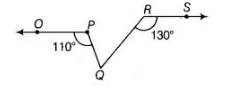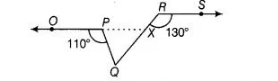# In the figure, if OP || RS, ∠OPQ = 110°`
Question:

In the figure, if OP || RS, ∠OPQ = 110° and ∠QRS = 130°, then ∠PQR is equal to(a) $40^{\circ}$

(b) $50^{\circ}$

(c) $60^{\circ}$

(d) $70^{\circ}$

Solution:

(c)

In the given figure, producing $O P$, to intersect $R Q$ at $X$.

Since, $O P \| R S$ and $R X$ is a transversal.

So, $\quad \angle R X P=\angle X R S \quad$ [alternate angles]$\Rightarrow$ $\angle R X P=130^{\circ}$ $\left[\because \angle Q R S=130^{\circ}\right.$ (given)]...(i)

Now, $R Q$ is a line segment.

So, $\angle P X Q+\angle R X P=180^{\circ}$ [linear pair axiom]

$\Rightarrow$ $\angle P X Q=180^{\circ}-\angle R X P=180^{\circ}-130^{\circ}$ [from Eq. (i)]

$\Rightarrow$ $\angle P X Q=50^{\circ}$

In $\triangle P Q X, \angle O P Q$ is an exterior angle,

$\therefore \quad \angle O P Q=\angle P X Q+\angle P Q X$

$[\because$ exterior angle $=$ sum of two opposite interior angles $]$

$\Rightarrow \quad 110^{\circ}=50^{\circ}+\angle P Q X$

$\Rightarrow \quad \angle P Q X=110^{\circ}-50^{\circ}$

$\therefore \quad \angle P Q R=60^{\circ} \quad[\because \angle P Q X=\angle P Q R]$